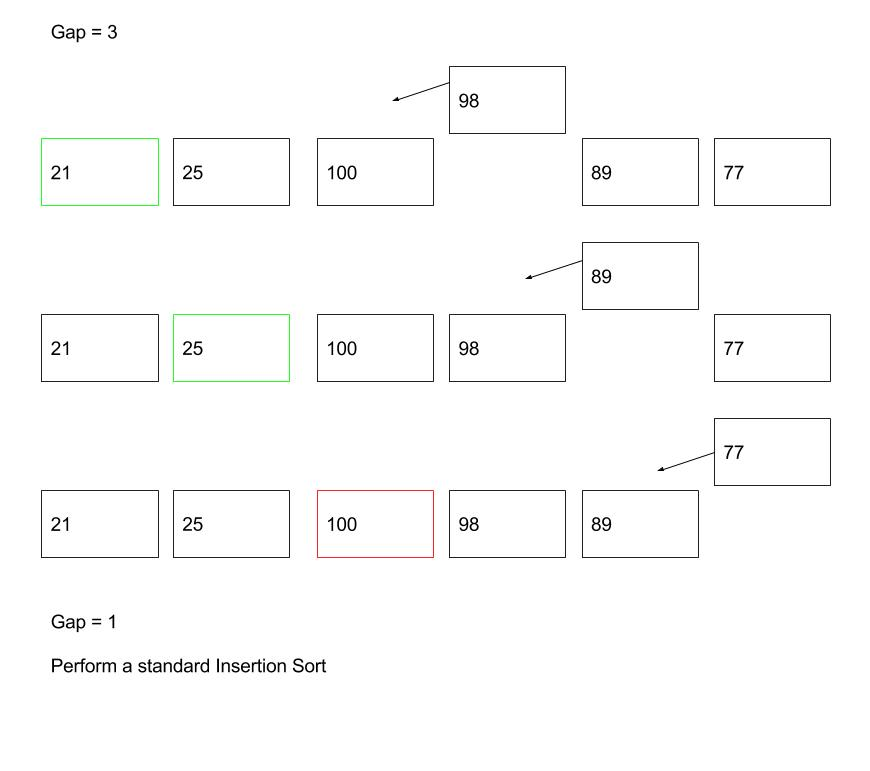### Data Structure and Algorithm

In this tutorial, we will learn a simple sorting algorithm - Shell Sort.

## Table Of Content

###### 1. Problem to Solve

Given a list of numbers as shown below, please sort them in ascending order.

Requirements:

• You are required to use Shell Sort algorithm when sorting the numbers.
• You are required to implement the algorithm in PHP language.
###### 2. Pseudocode

Shell Sort is a generalisation of Insertion Sort. Unlike Insertion Sort, instead of comparing contiguous items, it breaks the master list into several sub-lists using an interval i (which is known as the gap), then sorts the sub-lists with Insertion Sort.

We repeat the steps above until the gap becomes 1, then we essentially apply a standard Insertion Sort.

Apparently, the gap sequence plays an important role in this sort algorithm. Unfortunately, there is no perfect gap sequence, so to speak. Different researchers have come out a couple of different gap sequences, their performance depends heavily on the input data size.

Some popular gap sequences:

• Shell Sequence: FLOOR(N/2k)
• Pratt Sequence: 2p3q
• Knuth Sequence: (3k â€“ 1) / 2

We are using Shell Sequence in this tutorial.Pseudocode of Shell Sort algorithm can be written as follows:

###### 3. PHP Implementation

We need a FOR loop and a WHILE loop. We are using the FOR loop to iterate the master list and the WHILE loop to locate the position to insert the item.

The implementation is similar to Insertion Sort, except that we are comparing items that are \$gap apart until the \$gap values become 1. Then we perform a standard Insertion Sort.